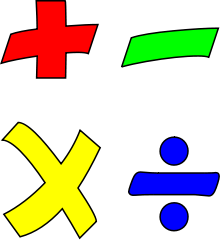# Reducing a Complex Algebraic FractionIn this lesson we are going to simplify a complex algebraic fraction.

First, here is the syntax we will use for algebraic expressions – so-called ‘one-line’ notation, that uses common keyboard operations such as ,-,*,/ and ^. If you find this syntax a little cumbersome, cut and paste any of these expressions to a ‘syntax converter’ located at this algebra resources page: http://www.algebra1help.com/links. It will create a nice looking textbook notation.

When we refer to a ‘complex’ fraction in algebra, we don’t really mean ‘complicated’, rather it is a fraction that has another fraction in its numerator or denominator (or both). So, here is our problem:

(x-y)^2/((x y)/(x^2-y^2))

Notice that the denominator is another fraction. The first thing we need to do is simplify the fraction in the denominator (there is nothing to simplify in the numerator). We start by factoring the denominator (of the denominator):

(x-y)^2/((x y)/((x-y)*(x y)))

We have employed a simple ‘difference of two squares” identity. Notice that now one of the factors in the numerator, (x y) is also found in the denominator. That means that we can reduce them. Since that was the only factor in the numerator, we replace it by “1”:

(x-y)^2/(1/(x-y))

Now we are going to replace the main fraction line with the division symbol : (imagine that there is a line between the dots!)

(x-y)^2:(1/(x-y))

Once we got rid of the complex fraction, we can convert the division into multiplication. This is done by ‘flipping’ the numerator and denominator of the second fraction according to the following rule a/b:c/d=a/b*d/c. Since in our case “c” is equal to 1, we can simply leave it out, once it becomes denominator:

(x-y)^2*(x-y)

The rest is a simple multiplication problem – we just need to add the numeric exponents (“1” is implied):

(x-y)^(2 1)

Which gives us the final result:

(x-y)^3

This wasn’t that ‘complex’, was it?

Stay tuned for more simple algebra lessons!